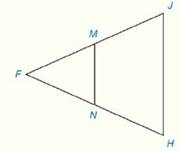Chapter 4.CR, Problem 24CRElementary Geometry For College St...

7th Edition
Alexander + 2 others
ISBN: 9781337614085

Solutions

Chapter
SectionElementary Geometry For College St...

7th Edition
Alexander + 2 others
ISBN: 9781337614085
Textbook Problem

Review ExercisesIn Review Exercises 22 to 24, M and N are the midpoints of F J - and F H - respectively.Given: M N = x 2 + 6 J H = 2 x ( x + 2 ) Prove: x ,   M N ,   J H

To determine

To Find:

The length of the line joining the mid points of the sides of the triangle and measure of sides based on the information provided.

Explanation

Formula Used:

Sum of the interior angle of a triangle is 180°.

Theorem on triangle:

The line segment that joins the midpoints of two sides of a triangle is parallel to the third side and has a length equal to one-half the length of the third side.

That is, ABC with midpoints M and N of AB- and AC-, respectively. Then MN-BC-. It can be concluded that BC=12(MN)

Calculation:

It is given that FJH is a triangle with M and N are the midpoints of FJ- and FH-.

Also given that MN=x2+6 and JH=2x(x+2)

Still sussing out bartleby?

Check out a sample textbook solution.

See a sample solution

The Solution to Your Study Problems

Bartleby provides explanations to thousands of textbook problems written by our experts, many with advanced degrees!

Get Started

Evaluate each expression: 4+232

Elementary Technical Mathematics

Evaluate the integrals in Problems 1-32. Identify the formula used. 2.

Mathematical Applications for the Management, Life, and Social Sciences

Let x be the function defined by s(t)=2tt21. Find s(4), s(0), s(a). s(2 + a), and s(t + 1).

Applied Calculus for the Managerial, Life, and Social Sciences: A Brief Approach

The graph of θ = 2 in polar coordinates is a: circle line spiral 3-leaved rose

Study Guide for Stewart's Single Variable Calculus: Early Transcendentals, 8th